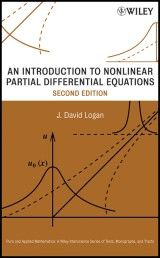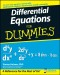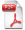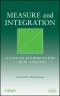# Details## An Introduction to Nonlinear Partial Differential Equations

Pure and Applied Mathematics: A Wiley Series of Texts, Monographs and Tracts, Band 89 2. Aufl.
 von: J. David Logan 133,99 € Verlag: Wiley Format: PDF Veröffentl.: 30.04.2008 ISBN/EAN: 9780470287088 Sprache: englisch Anzahl Seiten: 416

DRM-geschütztes eBook, Sie benötigen z.B. Adobe Digital Editions und eine Adobe ID zum Lesen.

## Beschreibungen

#### Titelbeschreibung

Praise for the First Edition: <p>"This book is well conceived and well written. The author has succeeded in producing a text on nonlinear PDEs that is not only quite readable but also accessible to students from diverse backgrounds."<br /> —SIAM Review</p> <p>A practical introduction to nonlinear PDEs and their real-world applications</p> <p>Now in a Second Edition, this popular book on nonlinear partial differential equations (PDEs) contains expanded coverage on the central topics of applied mathematics in an elementary, highly readable format and is accessible to students and researchers in the field of pure and applied mathematics. This book provides a new focus on the increasing use of mathematical applications in the life sciences, while also addressing key topics such as linear PDEs, first-order nonlinear PDEs, classical and weak solutions, shocks, hyperbolic systems, nonlinear diffusion, and elliptic equations. Unlike comparable books that typically only use formal proofs and theory to demonstrate results, An Introduction to Nonlinear Partial Differential Equations, Second Edition takes a more practical approach to nonlinear PDEs by emphasizing how the results are used, why they are important, and how they are applied to real problems.</p> <p>The intertwining relationship between mathematics and physical phenomena is discovered using detailed examples of applications across various areas such as biology, combustion, traffic flow, heat transfer, fluid mechanics, quantum mechanics, and the chemical reactor theory. New features of the Second Edition also include:</p> <ul> <li> <p>Additional intermediate-level exercises that facilitate the development of advanced problem-solving skills</p> </li> <li> <p>New applications in the biological sciences, including age-structure, pattern formation, and the propagation of diseases</p> </li> <li> <p>An expanded bibliography that facilitates further investigation into specialized topics</p> </li> </ul> <p>With individual, self-contained chapters and a broad scope of coverage that offers instructors the flexibility to design courses to meet specific objectives, An Introduction to Nonlinear Partial Differential Equations, Second Edition is an ideal text for applied mathematics courses at the upper-undergraduate and graduate levels. It also serves as a valuable resource for researchers and professionals in the fields of mathematics, biology, engineering, and physics who would like to further their knowledge of PDEs.</p>

#### Inhaltsverzeichnis

<p>Preface xi</p> <p><b>1. Introduction to Partial Differential Equations 1</b></p> <p>1.1 Partial Differential Equations 2</p> <p>1.1.1 Equations and Solutions 2</p> <p>1.1.2 Classification 5</p> <p>1.1.3 Linear versus Nonlinear 8</p> <p>1.1.4 Linear Equations 11</p> <p>1.2 Conservation Laws 20</p> <p>1.2.1 One Dimension 20</p> <p>1.2.2 Higher Dimensions 23</p> <p>1.3 Constitutive Relations 25</p> <p>1.4 Initial and Boundary Value Problems 35</p> <p>1.5 Waves 45</p> <p>1.5.1 Traveling Waves 45</p> <p>1.5.2 Plane Waves 50</p> <p>1.5.3 Plane Waves and Transforms 52</p> <p>1.5.4 Nonlinear Dispersion 54</p> <p><b>2. First-Order Equations and Characteristics 61</b></p> <p>2.1 Linear First-Order Equations 62</p> <p>2.1.1 Advection Equation 62</p> <p>2.1.2 Variable Coefficients 64</p> <p>2.2 Nonlinear Equations 68</p> <p>2.3 Quasilinear Equations 72</p> <p>2.3.1 The General Solution 76</p> <p>2.4 Propagation of Singularities 81</p> <p>2.5 General First-Order Equation 86</p> <p>2.5.1 Complete Integral 91</p> <p>2.6 A Uniqueness Result 94</p> <p>2.7 Models in Biology 96</p> <p>2.7.1 Age Structure 96</p> <p>2.7.2 Structured Predator-Prey Model 101</p> <p>2.7.3 Chemotherapy 103</p> <p>2.7.4 Mass Structure 105</p> <p>2.7.5 Size-Dependent Predation 106</p> <p><b>3. Weak Solutions to Hyperbolic Equations 113</b></p> <p>3.1 Discontinuous Solutions 114</p> <p>3.2 Jump Conditions 116</p> <p>3.2.1 Rarefaction Waves 118</p> <p>3.2.2 Shock Propagation 119</p> <p>3.3 Shock Formation 125</p> <p>3.4 Applications 131</p> <p>3.4.1 Traffic Flow 132</p> <p>3.4.2 Plug Flow Chemical Reactors 136</p> <p>3.5 Weak Solutions: A Formal Approach 140</p> <p>3.6 Asymptotic Behavior of Shocks 148</p> <p>3.6.1 Equal-Area Principle 148</p> <p>3.6.2 Shock Fitting 152</p> <p>3.6.3 Asymptotic Behavior 154</p> <p><b>4. Hyperbolic Systems 159</b></p> <p>4.1 Shallow-Water Waves: Gas Dynamics 160</p> <p>4.1.1 Shallow-Water Waves160</p> <p>4.1.2 Small-Amplitude Approximation 163</p> <p>4.1.3 Gas Dynamics 164</p> <p>4.2 Hyperbolic Systems and Characteristics 169</p> <p>4.2.1 Classification 170</p> <p>4.3 The Riemann Method 179</p> <p>4.3.1 Jump Conditions for Systems 179</p> <p>4.3.2 Breaking Dam Problem 181</p> <p>4.3.3 Receding Wall Problem 183</p> <p>4.3.4 Formation of a Bore 187</p> <p>4.3.5 Gas Dynamics 190</p> <p>4.4 Hodographs and Wavefronts 192</p> <p>4.4.1 Hodograph Transformation 192</p> <p>4.4.2 Wavefront Expansions 193</p> <p>4.5 Weakly Nonlinear Approximations 201</p> <p>4.5.1 Derivation of Burgers‘ Equation 202</p> <p><b>5.</b> Diffusion Processes 209</p> <p>5.1 Diffusion and Random Motion 210</p> <p>5.2 Similarity Methods 217</p> <p>5.3 Nonlinear Diffusion Models 224</p> <p>5.4 Reaction-Diffusion: Fisher’s Equation 234</p> <p>5.4.1 Traveling Wave Solutions 235</p> <p>5.4.2 Perturbation Solution 238</p> <p>5.4.3 Stability of Traveling Waves 240</p> <p>5.4.4 Nagumo‘s Equation 242</p> <p>5.5 Advection-Diffusion: Burgers’ Equation 245</p> <p>5.5.1 Traveling Wave Solution 246</p> <p>5.5.2 Initial Value Problem 247</p> <p>5.6 Asymptotic Solution to Burgers’ Equation 250</p> <p>5.6.1 Evolution of a Point Source 252</p> <p>Appendix: Dynamical Systems 257</p> <p><b>6. Reaction-Diffusion Systems 267</b></p> <p>6.1 Reaction-Diffusion Models 268</p> <p>6.1.1 Predator-Prey Model 270</p> <p>6.1.2 Combustion 271</p> <p>6.1.3 Chemotaxis 274</p> <p>6.2 Waveling IJ1Bve Solutions 277</p> <p>6.2.1 Model for the Spread of a Disease 278</p> <p>6.2.2 Contaminant Transport in Groundwater 284</p> <p>6.3 Existence of Solutions 292</p> <p>6.3.1 Fixed-Point Iteration 293</p> <p>6.3.2 Semilinear Equations 297</p> <p>6.3.3 Normed Linear Spaces 300</p> <p>6.3.4 General Existence Theorem 303</p> <p>6.4 Maximum Principles and Comparison Theorems 309</p> <p>6.4.1 Maximum Principles 309</p> <p>6.4.2 Comparison Theorems 314</p> <p>6.5 Energy Estimates and Asymptotic Behavior 317</p> <p>6.5.1 Calculus Inequalities 318</p> <p>6.5.2 Energy Estimates 320</p> <p>6.5.3 Invariant Sets 326</p> <p>6.6 Pattern Formation 333</p> <p><b>7. Equilibrium Models 345</b></p> <p>7.1 Elliptic Models 346</p> <p>7.2 Theoretical Results 352</p> <p>7.2.1 Maximum Principle 353</p> <p>7.2.2 Existence Theorem 355</p> <p>7.3 Eigenvalue Problems 358</p> <p>7.3.1 Linear Eigenvalue Problems 358</p> <p>7.3.2 Nonlinear Eigenvalue Problems 361</p> <p>7.4 Stability and Bifurcation 364</p> <p>7.4.1 Ordinary Differential Equations 364</p> <p>7.4.2 Partial Differential Equations 368</p> <p>References 387</p> <p>Index 395</p>

#### Rezension

"This book is an ideal text for applied mathematics courses at the upper-undergraduate and graduate levels. It also serves as a valuable resource for researchers and professionals in the fields of mathematics, biology, engineering, and physics who would like to further their knowledge of PDEs." (<i>Mathematical Reviews</i>, 2009c)

#### Autorenportrait

<p><b>J. David Logan</b>, PhD, is Willa Cather Professor of Mathematics at the University of Nebraska–Lincoln. He has authored several texts on elementary differential equations and beginning partial differential equations, including Applied Mathematics, Third Edition, also published by Wiley. Dr. Logan's research interests include mathematical physics, combustion and detonation, hydrogeology, and mathematical biology.</p>

#### Back cover copy

<p>Praise for the First Edition:</p> <p>"This book is well conceived and well written. The author has succeeded in producing a text on nonlinear PDEs that is not only quite readable but also accessible to students from diverse backgrounds."<br /> —SIAM Review</p> <p>A practical introduction to nonlinear PDEs and their real-world applications</p> <p>Now in a Second Edition, this popular book on nonlinear partial differential equations (PDEs) contains expanded coverage on the central topics of applied mathematics in an elementary, highly readable format and is accessible to students and researchers in the field of pure and applied mathematics. This book provides a new focus on the increasing use of mathematical applications in the life sciences, while also addressing key topics such as linear PDEs, first-order nonlinear PDEs, classical and weak solutions, shocks, hyperbolic systems, nonlinear diffusion, and elliptic equations. Unlike comparable books that typically only use formal proofs and theory to demonstrate results, An Introduction to Nonlinear Partial Differential Equations, Second Edition takes a more practical approach to nonlinear PDEs by emphasizing how the results are used, why they are important, and how they are applied to real problems.</p> <p>The intertwining relationship between mathematics and physical phenomena is discovered using detailed examples of applications across various areas such as biology, combustion, traffic flow, heat transfer, fluid mechanics, quantum mechanics, and the chemical reactor theory. New features of the Second Edition also include:</p> <ul> <li> <p>Additional intermediate-level exercises that facilitate the development of advanced problem-solving skills</p> </li> <li> <p>New applications in the biological sciences, including age-structure, pattern formation, and the propagation of diseases</p> </li> <li> <p>An expanded bibliography that facilitates further investigation into specialized topics</p> </li> </ul> <p>With individual, self-contained chapters and a broad scope of coverage that offers instructors the flexibility to design courses to meet specific objectives, An Introduction to Nonlinear Partial Differential Equations, Second Edition is an ideal text for applied mathematics courses at the upper-undergraduate and graduate levels. It also serves as a valuable resource for researchers and professionals in the fields of mathematics, biology, engineering, and physics who would like to further their knowledge of PDEs.</p>

US

## Diese Produkte könnten Sie auch interessieren:Differential Equations For Dummies
von: Steven Holzner16,99 €Measure and Integration
von: Leonard F. Richardson92,99 €